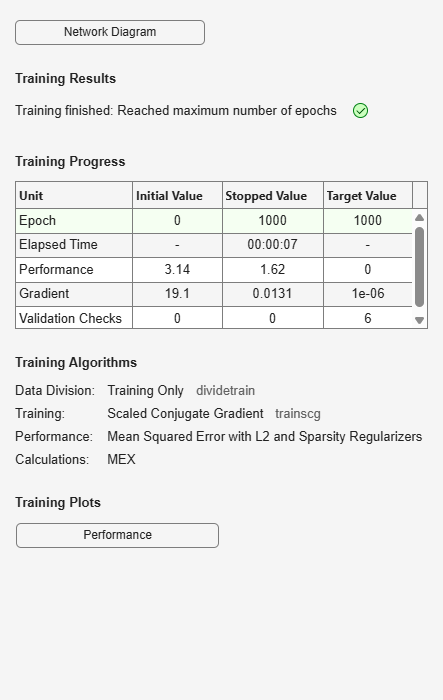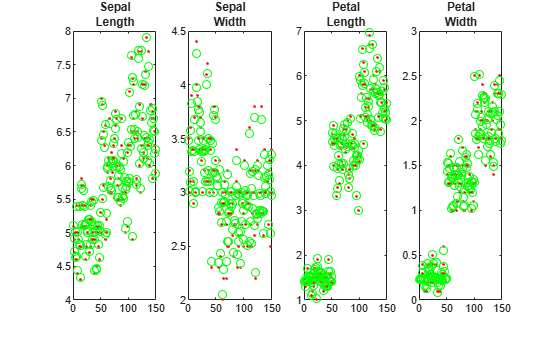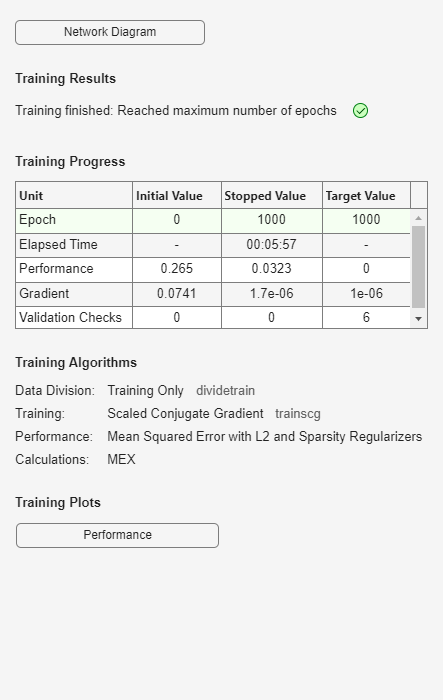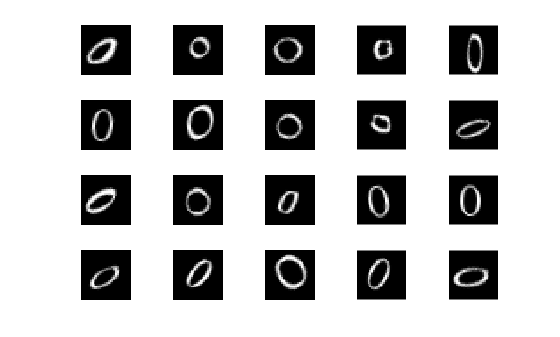# predict

Class: Autoencoder

Reconstruct the inputs using trained autoencoder

## Syntax

``Y = predict(autoenc,X)``

## Description

example

````Y = predict(autoenc,X)` returns the predictions `Y` for the input data `X`, using the autoencoder `autoenc`. The result `Y` is a reconstruction of `X`.```

## Input Arguments

expand all

Trained autoencoder, returned as an object of the `Autoencoder` class.

Input data, specified as a matrix of samples, a cell array of image data, or an array of single image data.

If the autoencoder `autoenc` was trained on a matrix, where each column represents a single sample, then `Xnew` must be a matrix, where each column represents a single sample.

If the autoencoder `autoenc` was trained on a cell array of images, then `Xnew` must either be a cell array of image data or an array of single image data.

Data Types: `single` | `double` | `cell`

## Output Arguments

expand all

Predictions for the input data `Xnew`, returned as a matrix or a cell array of image data.

• If `Xnew` is a matrix, then `Y` is also a matrix, where each column corresponds to a single sample (observation or example).

• If `Xnew` is a cell array of image data, then `Y` is also a cell array of image data, where each cell contains the data for a single image.

• If `Xnew` is an array of a single image data, then `Y` is also an array of a single image data.

## Examples

expand all

Load the training data.

`X = iris_dataset;`

The training data contains measurements on four attributes of iris flowers: Sepal length, sepal width, petal length, petal width.

Train an autoencoder on the training data using the positive saturating linear transfer function in the encoder and linear transfer function in the decoder.

```autoenc = trainAutoencoder(X,'EncoderTransferFunction',... 'satlin','DecoderTransferFunction','purelin');```Reconstruct the measurements using the trained network, `autoenc`.

`xReconstructed = predict(autoenc,X);`

Plot the predicted measurement values along with the actual values in the training dataset.

```for i = 1:4 h(i) = subplot(1,4,i); plot(X(i,:),'r.'); hold on plot(xReconstructed(i,:),'go'); hold off; end title(h(1),{'Sepal';'Length'}); title(h(2),{'Sepal';'Width'}); title(h(3),{'Petal';'Length'}); title(h(4),{'Petal';'Width'});```The red dots represent the training data and the green circles represent the reconstructed data.

Load the training data.

```XTrain = digitTrainCellArrayData; ```

The training data is a 1-by-5000 cell array, where each cell containing a 28-by-28 matrix representing a synthetic image of a handwritten digit.

Train an autoencoder with a hidden layer containing 25 neurons.

```hiddenSize = 25; autoenc = trainAutoencoder(XTrain,hiddenSize,... 'L2WeightRegularization',0.004,... 'SparsityRegularization',4,... 'SparsityProportion',0.15); ```

Load the test data.

```XTest = digitTestCellArrayData; ```

The test data is a 1-by-5000 cell array, with each cell containing a 28-by-28 matrix representing a synthetic image of a handwritten digit.

Reconstruct the test image data using the trained autoencoder, `autoenc`.

```xReconstructed = predict(autoenc,XTest); ```

View the actual test data.

```figure; for i = 1:20 subplot(4,5,i); imshow(XTest{i}); end ```View the reconstructed test data.

```figure; for i = 1:20 subplot(4,5,i); imshow(xReconstructed{i}); end ```## Version History

Introduced in R2015b